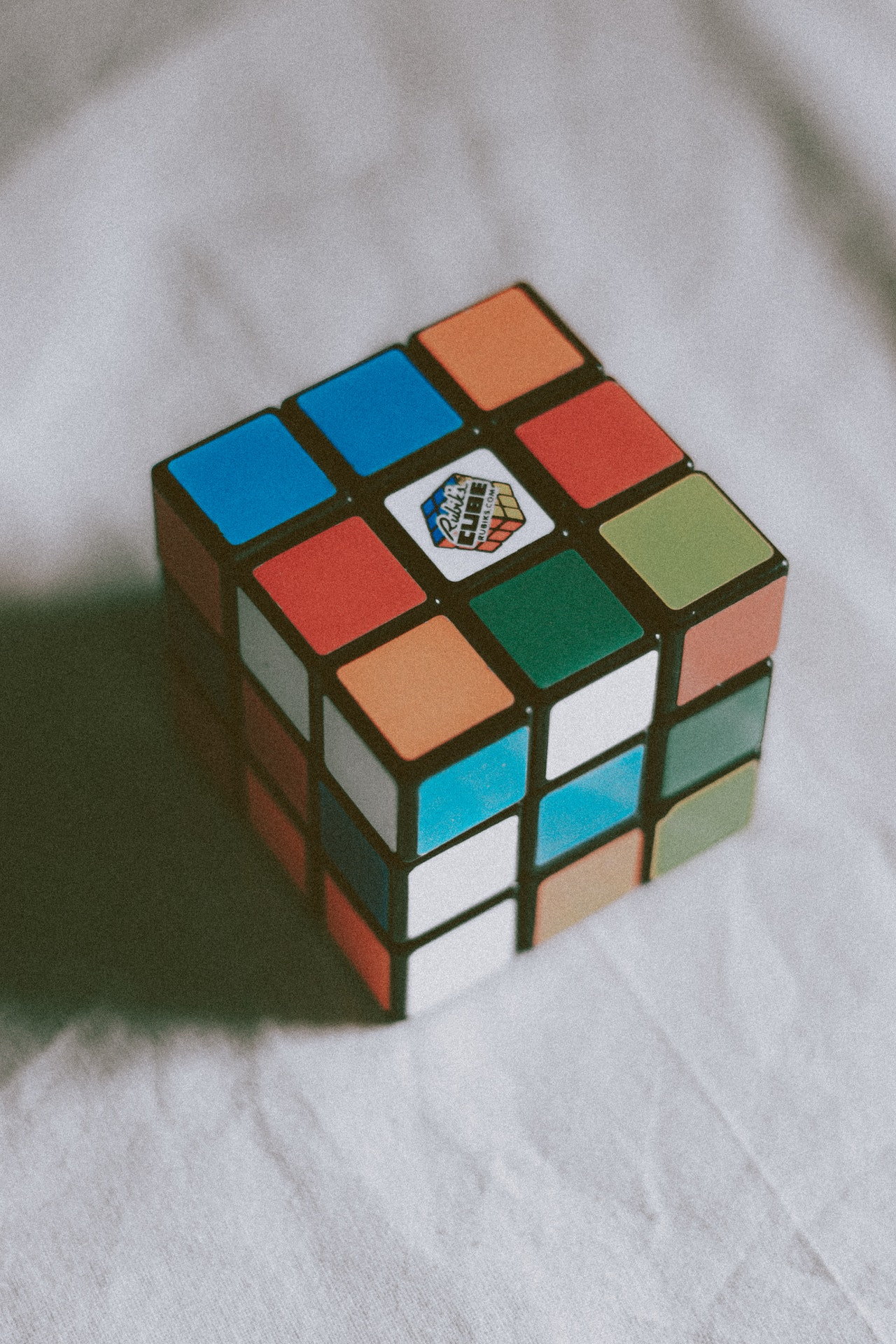# Permutation and Combination – DefinitionPermutation and combination are very important concepts in statistical calculations. The permutation is an arrangement of objects in a particular order by taking some or all objects at a time from a set of objects. The order of arrangement is important in the case of permutations. The combination is the selection of elements from a set where the order of elements is not relevant but only the selection matters. The permutation and the combination are two different ways of representing a group of objects or data. They refer to the various ways in which elements can be selected to form subsets from a set of objects, numbers, or observations taking part or all at a time.

## Difference Between Permutation and Combination

In permutation and combination, the same element can be repeated in the set of selected elements or may not be allowed to repeat. The basic difference between permutation and combination is that the permutation refers to the arrangement of selected elements into some sequence whereas combination refers to how many combinations of elements can be possible from a set of elements without considering the sequence of elements. In a set of given objects, the rearrangement of elements in different orders is called the process of permutation. The combination gives the counting of the number of combinations of elements without considering the order. Permutations can be used for things of different kinds and combinations are used for things of a similar kind.

## Example

For example, there are five digits A, B, C, D, and E. If we arrange these digits taking two at a time, there will be 20 possible outcomes such as AB, BC, BA, AC, and so on. These different possible selections are called permutations the value of which is 20.

Now, in the case of combinations, we have to find the subsets produced by taking two from the set of five elements without ordering. Now there can be selections where the same two elements are selected but in a different order which are considered as a single selection in case of combination.

For example, the AB and BA are considered as one selection. So there will be only 10 different combinations of elements taking two elements at a time.

## Formula of Permutation and Combination

• The permutation P of ‘n’ objects taken ‘k’ at a time is obtained by factorial n divided by the factorial of (n-k).
• The combination C of ‘n’ objects taken ‘k’ at a time is obtained by dividing the value of permutation (P) by factorial of k.
• The formula of permutation and combination directly gives the number of possible permutations and combinations without making any list of them.

## Learn Mathematics

Mathematics learning is quite different from learning other subjects because one needs to understand the conceptual things in mathematics without just memorizing. This learning helps to develop the analytical and logical approach in students while handling simple to complex mathematical problems. This is necessary to build a strong foundation in mathematics so students should focus on building conceptual clarity on mathematics topics. The teachers and mentors must focus on practice-based learning to develop problem-solving skills in students through some simple techniques that can be learned and applied easily. Regular practice helps students to build up the confidence to handle a variety of mathematical problems. The teachers must structure their teaching methodologies to make students more engaged in learning by developing a sense of interest. Through regular practice and problem-solving assignments, students become aware of their weak points and teachers guide them to improve on those areas to help them get better marks in exams. The online learning platforms of today are providing access to various tools and techniques that help to learn math more conveniently and effectively for students.